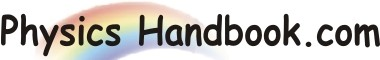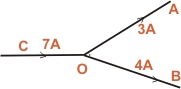HOME TOPICS DEFINITIONS TABLES LAWS INVENTIONS EXPERIMENTS QUIZ VIDEOS
 A B C D E F G H I J K L M N O P Q R S T U V W X Y Z
Electric Current (Scalar Quantity)
(I) Electric current I = q/t. As both charge and time are scalars, therefore electric current is a scalar quantity.(II) We show electric current in a wire by an arrow to indicate the direction of flow of positive charge. But such arrows are not vectors because they do not obey the laws of vector algebra. This point can be explained y referring to fig. The wire OA and OB carry currents of 3A and 4A respectively. The total current in the wire CO is 3 + 4 = 7A irrespective of the angle between the wires OA and OB. This is not surprising because the charge is conserved so that magnitudes of current in wires OA and OB must add to give the magnitude of current in wire CO.